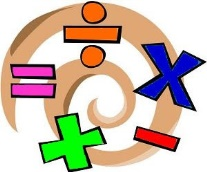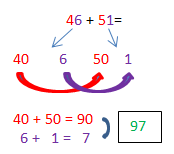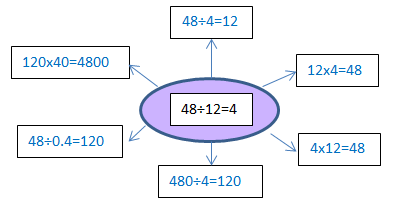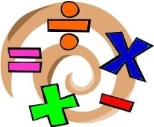Maths cornerMaths Talk for Ten

You may have heard your child(ren) talking about T410 (talk for ten) in maths lessons but not really know what it means.  Please don’t worry.  It is not a new area of maths you haven’t heard of before.  Instead, it is dedicated discussion time (10 minutes) within a maths lesson that we use with the aim of helping your child(ren) further understand some core areas of maths and develop their mathematical thinking and reasoning.

Format

In T410 discussions, children are encouraged to explore different ways to solve questions with the emphasis being on how they would tackle the problem rather than on reaching an accurate answer.  This means everyone can contribute a strategy and explain their thinking without worrying about actually calculating the answer.

Examples

Here are some examples of the sort of questions being explored in T410.

• How many different approaches could you use to solve 46+51=?
• Children could recognise that 51 is nearly 50 so they could move the one unit from the 51 and add it to the 46 changing the calculation to 47+50= which is easier t do mentally/
• They might also partition the question into tens and ones, add those separately then combine them at the end making the calculation 40+50=90 add 6+1=7.
•• I know that 18x3=54.  How can I use this to find out what 19x3=?
• Focusing on the questions, ‘What do you notice? What is the same? What is different?’ children can identify that the function is the same (x) and one of the numbers is the same (3) and that the format of the question is the same.  The difference is that the 18 in the first calculation has changed to 19.  Children then notice that 19 is one more than 18, so they need one more lot of 3 to find the answer.
• What methods could you use to solve this calculation?  45-39=
• Children could suggest using column methods, counting on/back, using a number line, partitioning, using concrete resources (cubes / dienes / counters etc.), pattern spotting or any other method that works for them.
• If I know 48÷12=4 what else do I know?
• This could link into families of facts: 48÷4=12, 12x4=48, 4x12=48
• Children could use their understanding of place value to increase / decrease part or all of the calculation by powers of 10: 480÷4=120, 120x40=4800, 48÷0.4=120 etc.Benefits

By taking the pressure of finding the answer away, everyone gets involved and has a go and discussing how they could tackle the question.  Being the fastest to answer a question is not valued in T410.  Rather, it is the thought process behind the maths that is important.  We have seen children sharing ideas and approaches in T410 that others then use with success later that lesson or in several lessons time.  Their ability to explain their mathematical reasoning is also helped by not focussing on the answer which should in turn help them build a deeper understanding of maths itself.

How can you get involved?

• Play games with your child(ren) that involves using numbers such as Uno or Cribb.  Ask them what they notice about the numbers they need to add – can they think of a good strategy to add them quickly and efficiently?
• Ask your child(ren) to tell you what else they know linked to a fact in the times table they are practising to help with the link between multiplication and division.
• If they are set a maths homework, ask them to explain to you what they are doing and why – they love telling you what to do and being the expert!

Questions?

If you have any questions, please drop me a line at the usual address: BJUenquiries@backwellfederation.co.uk